# Determination of Electron Flows

Making use of Equations (2.169), (2.177), (2.196), and (2.203), we obtain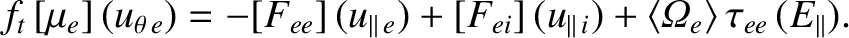(2.233)

It follows from Equations (2.183) and (2.233) that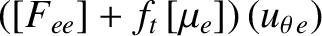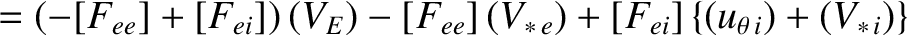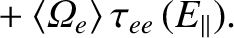(2.234)

However,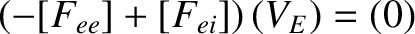because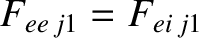, for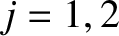, and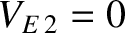. Hence, we get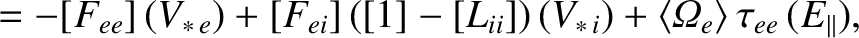(2.235)

where use has been made of Equation (2.214). It follows that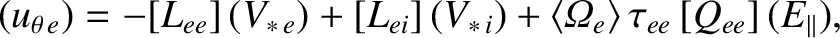(2.236)

where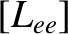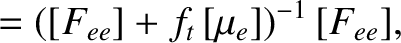(2.237)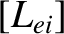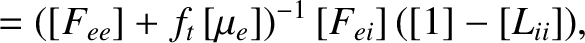(2.238)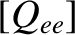(2.239)

Making use of Equations (2.197), (2.198), and (2.216), and only retaining terms up to first order in the small parameter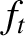, we get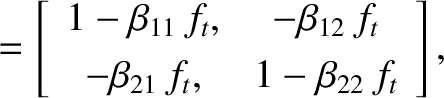(2.240)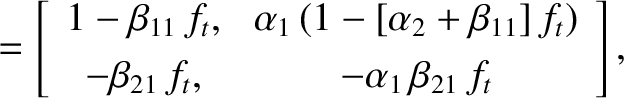(2.241)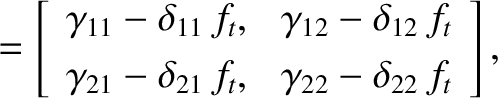(2.242)

where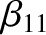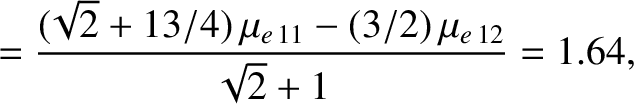(2.243)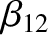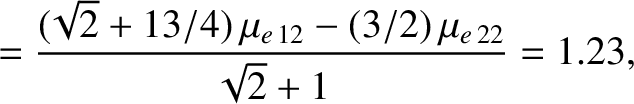(2.244)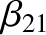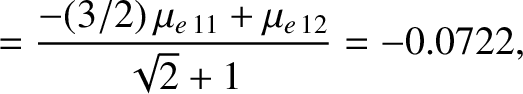(2.245)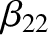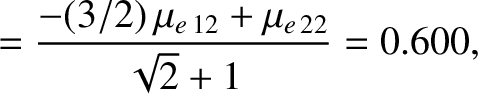(2.246)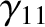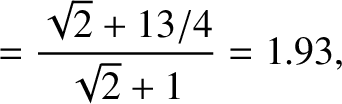(2.247)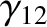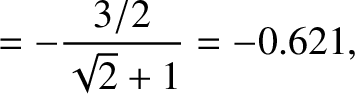(2.248)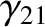(2.249)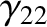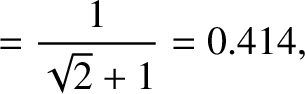(2.250)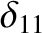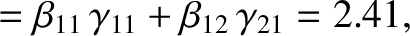(2.251)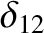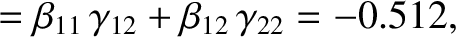(2.252)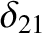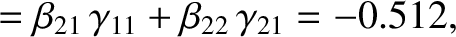(2.253)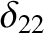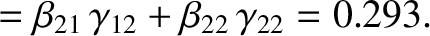(2.254)

Here use has been made of Equations (2.209)–(2.211), (2.217), and (2.218).

It follows from Equations (2.165), (2.166), (2.170), (2.181)–(2.185), (2.236), and (2.240)–(2.242) that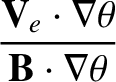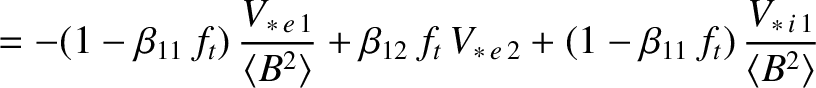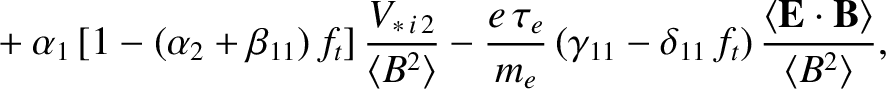(2.255)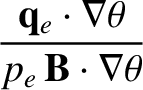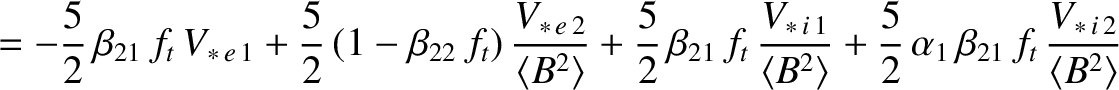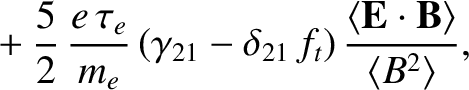(2.256)

and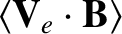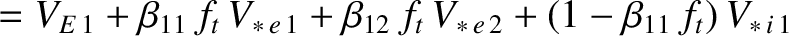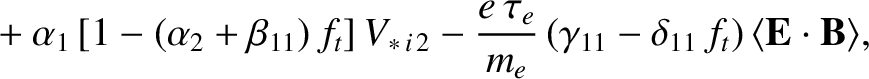(2.257)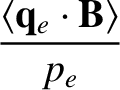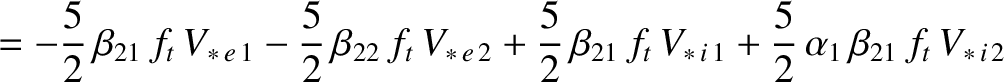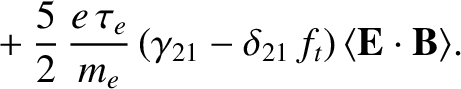(2.258)

Here, we have made use of the fact that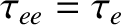.

In the circular magnetic flux-surface limit, Equations (2.255) and (2.256) reduce to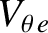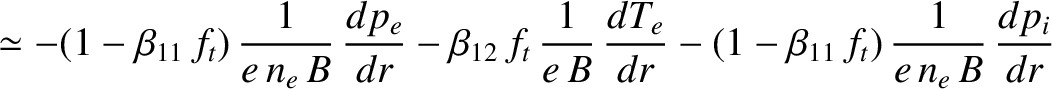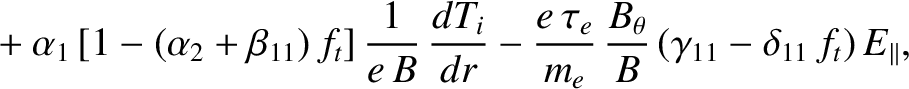(2.259)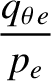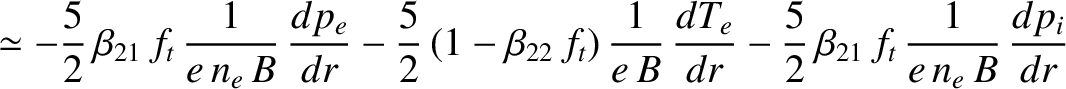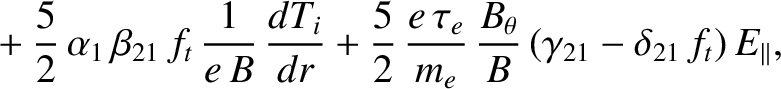(2.260)

whereas Equations (2.257)–(2.258) yield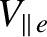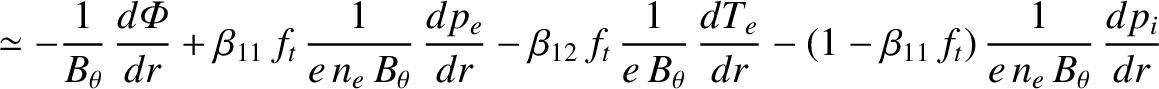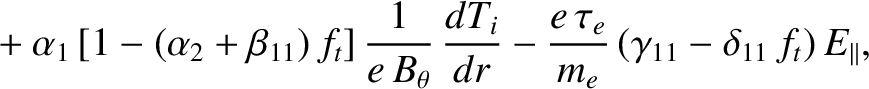(2.261)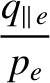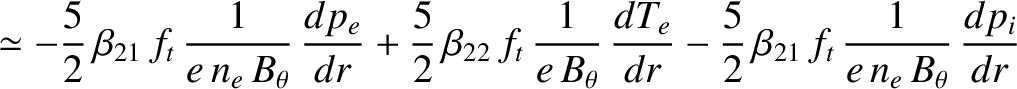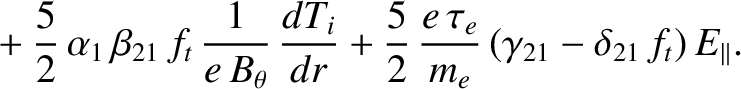(2.262)

Expressions (2.259)–(2.262) have many features in common with the corresponding expressions, (2.226)–(2.229), for the ions, despite being much more complicated in nature. In particular, the E-cross-B velocity makes no contribution to the poloidal component of the electron fluid velocity. Moreover, the poloidal component of the electron fluid velocity is similar in magnitude to a diamagnetic velocity, whereas the parallel component is larger by a factor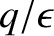.